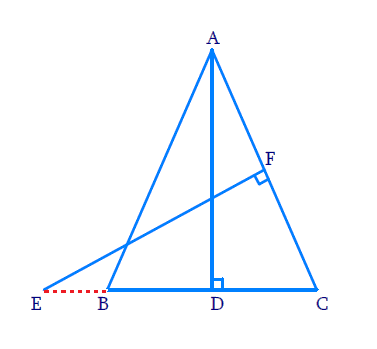# Ex.6.3 Q11 Triangles Solution - NCERT Maths Class 10

## Question

In Figure $$, E$$ is a point on side $$CB$$ produced of an isosceles $$\triangle ABC$$ with $$AB = AC$$. If $$AD \bot BC$$ and $$EF \bot AC$$, prove that $$\Delta A B D \sim \Delta E C F$$.

DiagramVideo Solution
Triangles
Ex 6.3 | Question 11

## Text Solution

Reasoning:

If two angles of one triangle are respectively equal to two angles of another triangle, then the two triangles are similar.

This is referred as the $$AA$$ criterion for two triangles.

Steps:

In $$\Delta ABD,\,\,\Delta ECF$$

\begin{align}&\angle ADB=\angle EFC={{90}^{\circ }}\\& (\because AD\bot BC \text{and}\,EF\bot AC) \\ &\angle ABD=\angle ECF \\& \begin{bmatrix}\because \text{In}\,\Delta ABC,AB=AC\\\Rightarrow \angle ABC=\angle ACB\end{bmatrix} \\&\Rightarrow \Delta ABD \sim \Delta ECF\\&\qquad(\text{AA}\,\,\text{criterion}) \\ \end{align}

Learn from the best math teachers and top your exams

• Live one on one classroom and doubt clearing
• Practice worksheets in and after class for conceptual clarity
• Personalized curriculum to keep up with school